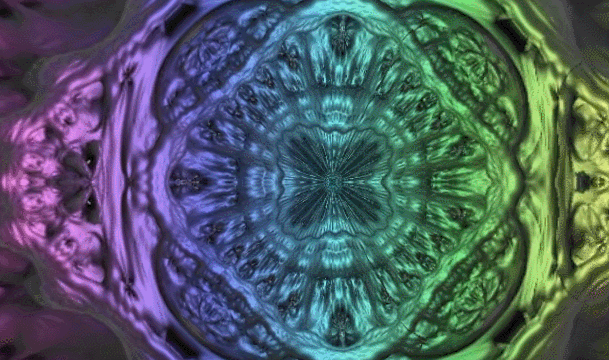# Fast Subsurface Scattering in Unity (Part 1)Most (if not all) optical phenomena that materials exhibit can be replicated by simulating how the individual rays of light propagate and interact. This approach is referred in the scientific literature as ray tracing, and it is often too computationally expensive for any real-time application. Most modern engines rely on massive simplifications that, despite being unable to reproduce photorealism, can produce a believable approximation. This tutorial introduces a fast, cheap and convincing solution that can be used to simulate translucent materials which exhibit subsurface scattering.

This is a two part series:

# The Mathematics of Diffraction GratingThis post introduces the mathematics behind the optical phenomenon known as diffraction grating, which is responsible for iridescent reflections in many materials.You can find the complete series here:

A link to download the Unity project used in this series is also provided at the end of the page.

# An Introduction to Gradient DescentThis post concludes the theoretical introduction to Inverse Kinematics, providing a programmatical solution based on gradient descent. This article does not aim to be a comprehensive guide on the topic, but a gentle introduction. The next post, Inverse Kinematics for Robotic Arms, will show an actual C# implementation of this algorithm in with Unity.The other post in this series can be found here:DistanceFromTarget

At the end of this post you can find a link to download all the assets and scenes necessary to replicate this tutorial.

# Implementing Forward KinematicsThis tutorial continues our quest to solve the problem of forward kinematics. After exploring a mathematical solution in The Mathematics of Forward Kinematics, we will see how to translate it into C# for Unity. The next tutorial, An Introduction to Gradient Descent, will finally show the theoretical foundations to solve inverse kinematics.The other post in this series can be found here:

At the end of this post you can find a link to download all the assets and scenes necessary to replicate this tutorial.

# The Mathematics of Forward KinematicsThis tutorial starts our journey into the world of inverse kinematics. There are countless ways to approach this problem, but they all starts with forward kinematics.

Inverse kinematics takes a point in space, and tells you how to move your arm to reach it. Forward kinematics solves the opposite, dual problem. Knowing how you are moving your arm, it tells which point in space it reaches.The other post in this series can be found here:

At the end of this post you can find a link to download all the assets and scenes necessary to replicate this tutorial.

# Positioning and TrilaterationThis post shows how it is possible to find the position of an object in space, using a technique called trilateration. The traditional approach to this problem relies on three measurements only. This tutorial addresses how to it is possible to take into account more measurements to improve the precision of the final result. This algorithm is robust and can work even with inaccurate measurements.

If you are unfamiliar with the concepts of latitude and longitude, I suggest you read the first post in this series: Understanding Geographical Coordinates.

# Understanding Geographical CoordinatesThis series introduces the concept of trilateration. This technique can be applied to a wide range of problems, from indoor localisation to earthquake detection. This first post provides a general introduction to the concept of geographical coordinates, and how they can be effectively manipulated. The second post in the series, Positioning and Trilateration, will cover the actual techniques used to identify the position of an object given independent distance readings. Most trilateration tutorials require the measures from the sensors to be precise and consistent. The approach here presented, instead, is highly robust and can tolerate inaccurate readings.

# Fractals 101: The Mandelbrot SetThis is the second part of Fractals 101, a series of tutorial dedicated to fractals. This post will investigate two popular fractals: the Mandelbrot set and its 3D cousin, the Mandelbulb.# The Autocorrelation FunctionThe purpose of this tutorial is to show a simple technique to estimate periodicity in time series, called autocorrelation.

This tutorial is part of a longer series that focuses on how to analyse time series.

# Time Series DecompositionThis tutorial will teach you how you can extract valuable information from time series, such as your sold copies on Steam or your Google Analytics. The previous part of this series introduced a technique called moving average, which has been used to attenuate the effects of noise in a signal. When signals represent an event that evolves over time, we are in front of a time series. Classical decomposition is a technique that attempts to find the main trends within time series.